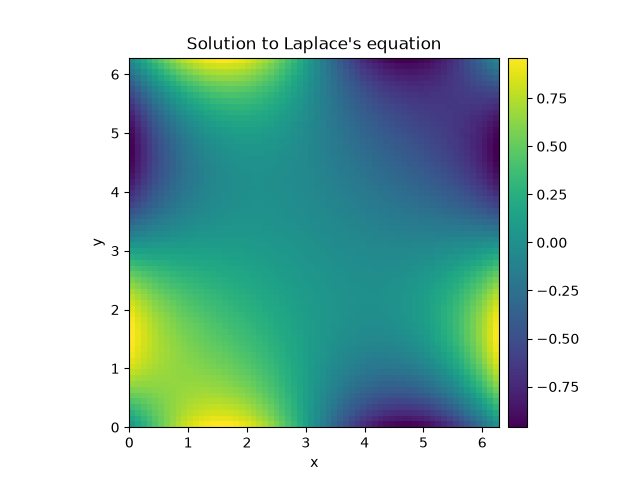# 2.2. Solving Laplace’s equation in 2d

This example shows how to solve a 2d Laplace equation with spatially varying boundary conditions.```import numpy as np

from pde import CartesianGrid, solve_laplace_equation

grid = CartesianGrid([[0, 2 * np.pi]] * 2, 64)
bcs = [{"value": "sin(y)"}, {"value": "sin(x)"}]

res = solve_laplace_equation(grid, bcs)
res.plot()
```

Total running time of the script: (0 minutes 0.781 seconds)